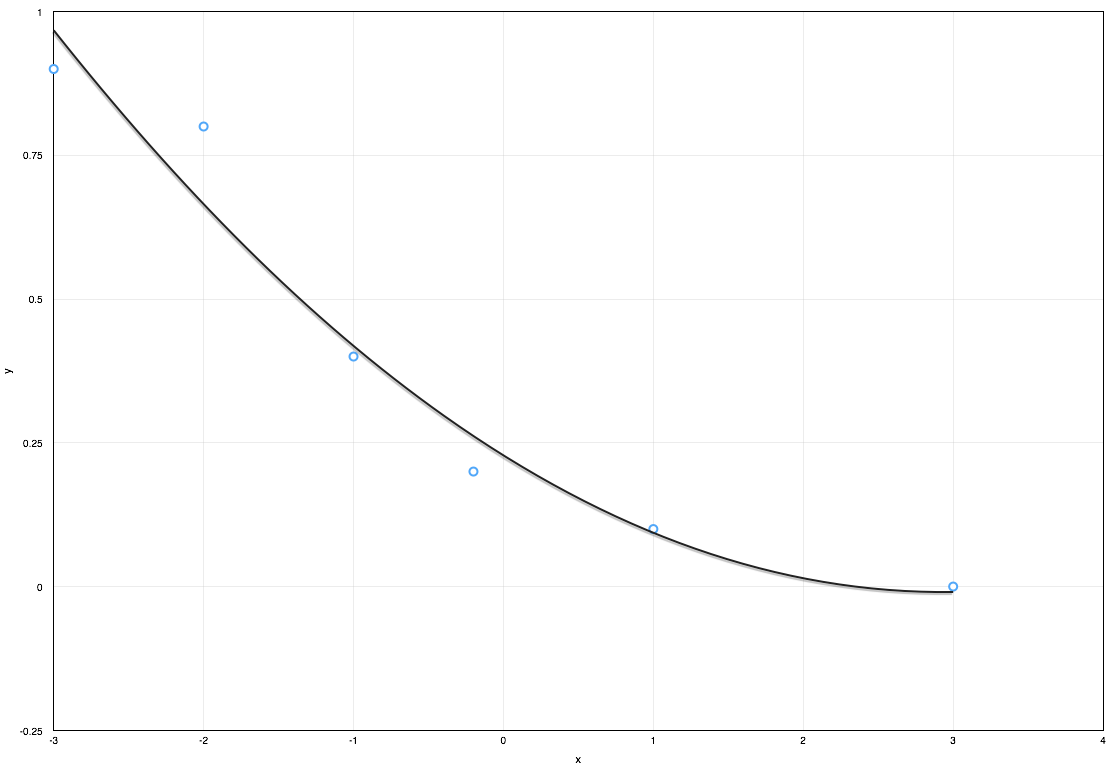f
Neutrium

# Algebra

Fitting of a Polynomial using Least Squares MethodApproximating a dataset using a polynomial equation is useful when conducting engineering calculations as it allows results to be quickly updated when inputs change without the need for manual lookup of the dataset. The most common method to generate a polynomial equation from a given data set is the least squares method. This article demonstrates how to generate a polynomial curve fit using the least squares method.

Exponent LawsAlgebraic expressions can often be simplified and subsequently solved through the use of the exponent laws (also called laws of indices or power laws). These laws allow an equation to be manipulated into a form which provides enhanced readability or opens up potential simplifications and substitutions. This article provides a reference for these laws.

Algebraic FactoringWhen developing algebraic expressions it is often useful to factorise the expression to familiar components. Through factorisation, one can increases both the readability and manipulability of the expression. This article summarises the common algebra factorisation relationships.